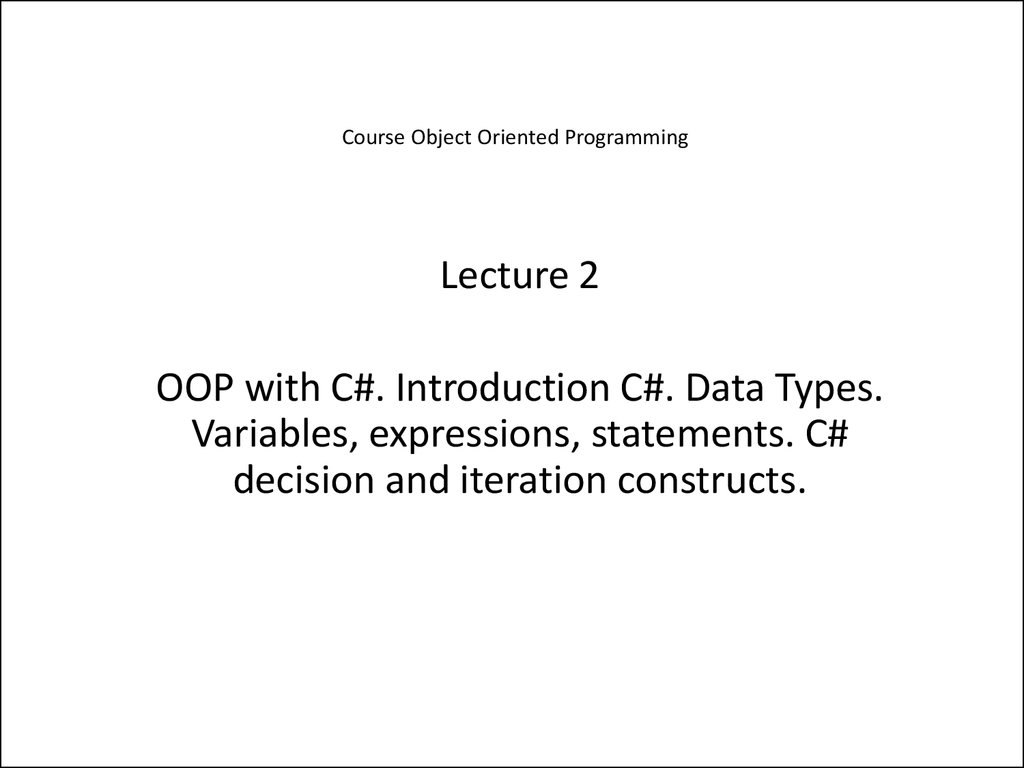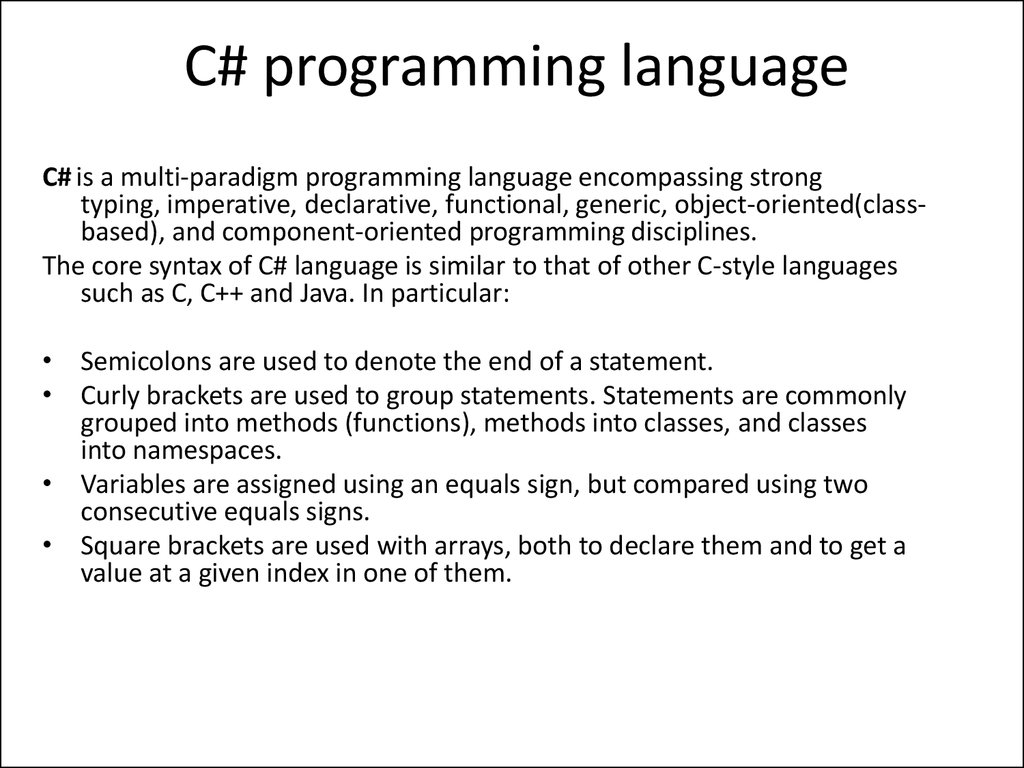# Course object oriented programming lecture 2

## 1. Course Object Oriented Programming

Lecture 2
OOP with C#. Introduction C#. Data Types.
Variables, expressions, statements. C#
decision and iteration constructs.

## 2. C# programming language

C# is a multi-paradigm programming language encompassing strong
typing, imperative, declarative, functional, generic, object-oriented(classbased), and component-oriented programming disciplines.
The core syntax of C# language is similar to that of other C-style languages
such as C, C++ and Java. In particular:
• Semicolons are used to denote the end of a statement.
• Curly brackets are used to group statements. Statements are commonly
grouped into methods (functions), methods into classes, and classes
into namespaces.
• Variables are assigned using an equals sign, but compared using two
consecutive equals signs.
• Square brackets are used with arrays, both to declare them and to get a
value at a given index in one of them.

## 3. Data Types

Data is the fundamental currency of the
computer. All computer processing deals with
analysis, manipulation and processing of data.
computers. It is not surprising then, to learn
that data is also fundamental to the C#
language.

## 4. Data Types supported by C#

C# is a strongly typed language, that is, every object or
entity you create in a program must have definite
type. This allows the compiler to know how big it is
(i.e. how much storage is required in memory) and
what it can do (i.e. and thereby make sure that the
programmer is not misusing it). There are thirteen
basic data types in C#, note that 1 byte equals 8 bits
and each bit can take one of two values (i.e. 0 or 1).

## 6. Variables

The memory locations used to store a program’s
data are referred to as variables because as the
program executes the values stored tend to
change.
Each variable has three aspects of interest, its:
1. type.
2. value.
The data type of a variable informs us of what type of data and what range of values
can be stored in the variable and the memory address tells us where in memory
the variable is located.

## 7. Declaration of Variables

Syntax: <type> <name>;
Example
int i;
char a, b, ch;
All statements in C# are terminated with a semi-colon.

## 8. Naming of Variables

The names of variables and functions in C# are commonly
called identifiers. There are a few rules to keep in mind
when naming variables:
1. The first character must be a letter or an underscore.
2. An identifier can consist of letters, numbers and
underscores only.
3. Reserved words (int, char, double, …) cannot be used
as variable names.
In addition, please note carefully that C# is case sensitive. For example, the
identifiers Rate, rate and RATE are all considered to be different by the C#
compiler.

## 9. Initialize during variable declaration

Syntax: type var_name = constant;
Example
int i = 20; //i declared and given the value 20
char ch = ‘a’//ch declared and initialised with value .a.
int i = 2, j = 4, k, l = 5; //i, j and l initialised, k not initialised
Declare first then assign
Example
int i, j, k; //declare
i = 2; //assign
j = 3;
k = 5;

## 11. Console Input/Output (I/O)

Output
Syntax:
Console.WriteLine(<control_string>,<optional
_other_arguments);
For example:
Console.WriteLine("Hello World!");

## 12. Console Input/Output (I/O)

int a = 2, b = 3, c = 0;
c=a+b;
Console.WriteLine("c has the value {0}", c);
Console.WriteLine("{0} + {1} = {2}", a, b, c);
Here the symbols {0}, {1} etc. are placeholders where the values
of the optional arguments are substituted.

## 13. Console Input/Output (I/O)

Input
The string before the method means that whatever the user types on the
keyboard is returned from the method call and presented as a string.
It is up to the programmer to retrieve that data. An example is:
string input = "";
int data = 0;
Console.WriteLine("Please enter an integer value: ");
Console.ReadLine(); //user input is stored in the string input.
data = Convert.ToInt32(input);
Console.WriteLine("You entered {0}", data);

## 14. Operators

A strong feature of C# is a very rich set of built in operators including
arithmetic, relational, logical and bitwise operators.
Assignment =
Syntax: <lhs> = <rhs>;
where lhs means left hand side and rhs means right hand side.
Example
int i, j, k;
i = 20; // value 20 assigned to variable i
i = (j = 25); /* in C#, expressions in parentheses are always evaluated
first, so j is assigned the value 25 and the result of this assignment (i.e.
25) is assigned to i */
i = j = k = 10;

## 15. Arithmetic Operators

Arithmetic Operators (+, -, *, /, %)
- subtraction
* multiplication
/ division
% modulus
+ and - have unary and binary forms, i.e. unary operators take only one
operand, whereas binary operators require two operands.
Example
x = -y; // unary subtraction operator
p = +x * y; // unary addition operator
x = a + b; // binary addition operator
y = x - a; // binary subtraction operator

## 16. Increment and Decrement operators (++, - -)

Increment (++) and decrement (- -) are unary operators which cause the value of the
variable they act upon to be incremented or decremented by 1 respectively. These
operators are shorthand for a very common programming task.
Example
x++; //is equivalent to x = x + 1;
++ and - - may be used in prefix or postfix positions, each with a different meaning. In
prefix usage the value of the expression is the value after incrementing or
decrementing. In postfix usage the value of the expression is the value before
incrementing or decrementing.
Example
int i, j = 2;
i = ++j; // both i and j have the value 3
i = j++; // now i = 3 and j = 4

## 17. Special Assignment Operators (+=, -=, *=, /=, %=, &=)

Special Assignment Operators
(+=, -=, *=, /=, %=, &=)
Example
x += i + j; // this is the same as x = x + (i + j);
These shorthand operators improve the speed of execution as they require
the expression and variable to be evaluated once rather than twice.

## 18. Statements

Expression Statements
x = 1;//simple statement
Console.WriteLine(.Hello World!.);//also statement
x = 2 + (3 * 5) – 23;//complex statement
Compound Statements or Blocks
{
statement
statement
statement
}

If statement

## 20.

Example
int numerator, denominator;
Console.WriteLine(“Enter two integer values for the numerator and
denominator”);
if (denominator != 0)
Console.WriteLine(“{0}/{1} = {2}”, numerator, denominator,
numerator/denominator);
else
Console.WriteLine(“Invalid operation can’t divide by 0”);

## 21.

The statement body can include more than one statement but make sure
they are group into a code block i.e. surrounded by curly braces.
Example
int x, y, tmp;
if ( x > y)
{
tmp = x;
x = y;
y = tmp;
}

## 22. Nested if Statement

Nested if statements occur when one if statement is
nested within another if statement.
Example
if (x > 0)
if ( x > 10)
Console.WriteLine(“x is greater than both 0 and 10”);
else
Console.WriteLine(“x is greater than 0 but less than or
equal to 10”);
else
Console.WriteLine(“x is less than or equal to 0”);

## 23. if - else - if operator

If a program requires a choice from one of many cases, successive if
statements can be joined together to form a if - else - if ladder.

## 25. Conditional Operator ?:

There is a special shorthand syntax that gives the same result
as
if (expression )
true_statement;
else
false_statement;
syntax: expression ? true_statement : false_statement;
The ?; requires three arguments and is thus ternary. The main
advantage of this operator is that it is succinct.

## 26.

Example
max = x >= y ? x : y;
which is the equivalent of
if ( x >= y)
max = x;
else
max = y;

## 27. Switch Statement

This statement is similar to the if-else-if ladder but is clearer, easier to code
and less error prone.

## 28.

Example
double num1, num2, result;
char op;
Console.WriteLine(“Enter number operator number \n”);
switch(op)
{
case “+”:
result = num1 + num2;
break;
case “-”:
result = num1 - num2;
break;
case “*”:
result = num1 * num2;
break;
case “/”:
if(num2 != 0)
{
result = num1 / num2;
break;
} //else fall through to error statement
default:
Console.WriteLine(“ERROR- invalid operation or divide by 0.0 \n”);
}
Console.WriteLine(“{0} {1},{2} = {3}\n”, num1, op, num2, result);

## 29. Iterative Statements

For statement
While statement
Do while statement
Break statement
Continue statement

## 30. The while Looping Constructs

The while looping construct is useful should you wish to execute a block of statements until some
terminating condition has been reached. Within the scope of a while loop, you will need to ensure
this terminating event is indeed established; otherwise, you will be stuck in an endless loop. In the
following example, the message “In while loop” will be continuously printed until the user
terminates the loop by entering yes at the command prompt:
static void ExecuteWhileLoop()
{
string userIsDone = "";
// Test on a lower-class copy of the string.
while(userIsDone.ToLower() != "yes")
{
Console.Write("Are you done? [yes] [no]: ");
Console.WriteLine("In while loop");
}
}

## 31. The do/while Looping Constructs

Closely related to the while loop is the do/while statement. Like a simple while
loop, do/while is used when you need to perform some action an undetermined
number of times. The difference is that do/while loops are guaranteed to execute
the corresponding block of code at least once. In contrast, it is possible that a
simple while loop may never execute if the terminating condition is false from the
onset.
static void ExecuteDoWhileLoop()
{
string userIsDone = "";
do
{
Console.WriteLine("In do/while loop");
Console.Write("Are you done? [yes] [no]: ");
}while(userIsDone.ToLower() != "yes"); // Note the semicolon!
}

## 32. Decision Constructs

• C# defines two simple constructs to alter the
flow of your program, based on various
contingencies:
• -The if/else statement
• -The switch statement

## 33.

• C# Relational and Equality Operators
• Logical operators

## 34. The if/else statement

• static void IfElseExample()
• {
• // This is illegal, given that Length returns an int, not a
bool.
• string stringData = "My textual data";
• if(stringData.Length)
• {
• Console.WriteLine("string is greater than 0
characters");
• }
• }

## 35. The switch Statement

// Switch on a numerical value.
static void ExecuteSwitch()
{
Console.WriteLine("1 [C#], 2 [VB]");
int n = int.Parse(langChoice);
switch (n)
{
case 1:
Console.WriteLine("Good choice, C# is a fine language.");
break;
case 2: